Courses

# Olympiad Test: Direction Sense Test - 2

## 15 Questions MCQ Test Mathematics Olympiad Class 7 | Olympiad Test: Direction Sense Test - 2

Description
This mock test of Olympiad Test: Direction Sense Test - 2 for Class 7 helps you for every Class 7 entrance exam. This contains 15 Multiple Choice Questions for Class 7 Olympiad Test: Direction Sense Test - 2 (mcq) to study with solutions a complete question bank. The solved questions answers in this Olympiad Test: Direction Sense Test - 2 quiz give you a good mix of easy questions and tough questions. Class 7 students definitely take this Olympiad Test: Direction Sense Test - 2 exercise for a better result in the exam. You can find other Olympiad Test: Direction Sense Test - 2 extra questions, long questions & short questions for Class 7 on EduRev as well by searching above.
QUESTION: 1

### Reena walked 10 feet from A to B in the East. Then she turned to the right and walked 3 feet. Again she turned to the right and walked 14 feet. How far is she from A?

Solution: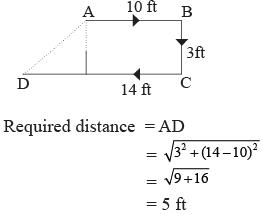QUESTION: 2

### If A × B means A is to the south of B; A + B means A is to the north of B; A % B means A is to the east of B; A - B means A is to the west of B; then in P % Q + R - S, S is in which direction with respect to Q?

Solution:

According to P % Q + R–S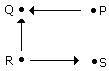S is in the South-East of Q.

QUESTION: 3

### One morning after sunrise, Vimal started to walk. During this walking he met Stephen who was coming from opposite direction. Vimal watched the shadow of Stephen to the right of him (Vimal). To Which direction Vimal was facing?

Solution:

Sun rises in the east. So the shadow of a man will always fall towards the west. Since the shadow of Stephen is to the right of Vimal. Hence Vimal is facing towards South.

QUESTION: 4

Golu started from his house towards North. After covering a distance of 8 km. he turned towards left and covered a distance of 6 km. What is the shortest distance now from his house?

Solution: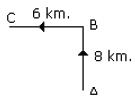QUESTION: 5

P started from his house towards west. After walking a distance of 25 m. He turned to the right and walked 10 m. He then again turned to the right and walked 15 m. After this he is to turn right at 135o and to cover 30 m. In which direction should he go?

Solution: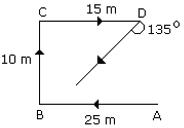Hence, he should go in the South-West direction.

QUESTION: 6

Rohit walked 25 m towards South. Then he turned to his left and walked 20 m. He then turned to his left and walked 25 m. He again turned to his right and walked 15 m. At what distance is he from the starting point and in which direction?

Solution: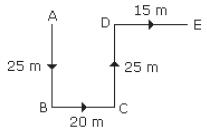Required distance = AE
= 20 + 15
= 35 m towards east.

QUESTION: 7

Umesh directly went from P to Q which is 9 feet distant. Then he turns to the right and walked 4 feet. After this he turned to the right and walked a distance which is equal from P to Q. Finally he turned to the right and walked 3 feet. How far is he now from P?

Solution: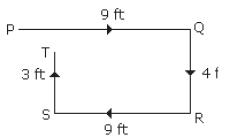Required distance = PT
= 4 – 3
= 1 ft.

QUESTION: 8

Ravi left home and cycled 10 km towards South, then turned right and cycled 5 km and then again turned right and cycled 10 km. After this he turned left and cycled 10 km. How many kilometers will he have to cycle to reach his home straight?

Solution: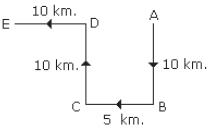Required distance = AE = 5 + 10
= 15 km.

QUESTION: 9

One morning after sunrise Nivedita and Niharika were talking to each other face to face at Dalphin crossing. If Niharika’s shadow was exactly to the right of Nivedita, Which direction Niharika was facing?

Solution: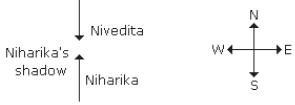In the morning the sun rises in the East. Hence any shadow falls in the West. Since Niharika shadow was exactly to the right of Nivedita. Hence, Nikharia is facing towards North.

QUESTION: 10

Shyam walks 5 km towards East and then turns left and walks 6 km. Again he turns right and walks 9 km. Finally he turns to his right and walks 6 km. How far is he from the starting point?

Solution: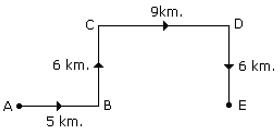Required distance
= AE = 5 + 9
= 14 km.

QUESTION: 11

X started to walk straight towards South. After walking 5 m he turned to the left and walked    3 m. After this he turned to the right and walked 5 m. Now to which direction X is facing?

Solution: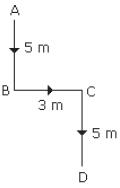Hence, X will face in the end towards South.

QUESTION: 12

A boy rides his bicycle northward, then turned left and rode 1 km and again turned left and rode 2 km. He found himself 1 km west of his starting point. How far did he ride northward initially?

Solution: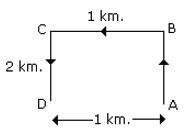The boy rides 2 km northward.

QUESTION: 13

Direction: Each of the following questions is based on the following information:​
1. A # B means B is at 1 metre to the right of A.
2. A \$ B means B is at 1 metre to the North of A.
3. A * B means B is at 1 metre to the left of A.
4. A @ B means B is at 1 metre to the south of A.
5. In each question the first person from the left is facing North.
Q. According to X @ B * P, P is in which direction with respect to X?

Solution:

According to X @ B * P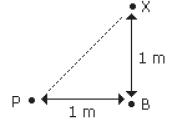Hence, P is in South-West of X.

QUESTION: 14

Direction: Each of the following questions is based on the following information:​
1. A # B means B is at 1 metre to the right of A.
2. A \$ B means B is at 1 metre to the North of A.
3. A * B means B is at 1 metre to the left of A.
4. A @ B means B is at 1 metre to the south of A.
5. In each question the first person from the left is facing North.
Q. According to M # N \$ T, T is in which direction with respect to M?

Solution:

According to M # N \$ T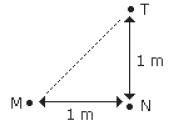Hence, T is in the North-East of M.

QUESTION: 15

Direction: Each of the following questions is based on the following information:​
1. A # B means B is at 1 metre to the right of A.
2. A \$ B means B is at 1 metre to the North of A.
3. A * B means B is at 1 metre to the left of A.
4. A @ B means B is at 1 metre to the south of A.
5. In each question the first person from the left is facing North.
Q. According to P # R \$ A * U, in which direction is U with respect to P?

Solution:

According to P # R \$ A * U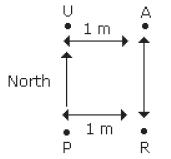Hence, U is in North direction with respect to P.